Updating search results...

# 28 Results

View
Selected filters:
• MCCRS.Math.Content.6.EE.B.7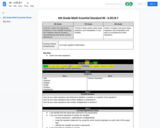Conditional Remix & Share Permitted
CC BY-NC-SA
Rating
0.0 stars

Solve one-step equations
Solve one-step linear equations in one variable involving non-negative rational numbers.
*(guaranteeing only whole numbers)

Subject:
Mathematics
Material Type:
Activity/Lab
Author:
Liberty Public Schools
04/12/2021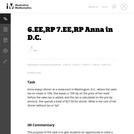Unrestricted Use
CC BY
Rating
0.0 stars

This is a task from the Illustrative Mathematics website that is one part of a complete illustration of the standard to which it is aligned. Each task has at least one solution and some commentary that addresses important asects of the task and its potential use. Here are the first few lines of the commentary for this task: Anna enjoys dinner at a restaurant in Washington, D.C., where the sales tax on meals is 10%. She leaves a 15% tip on the price of her meal before the s...

Subject:
Mathematics
Material Type:
Activity/Lab
Provider:
Illustrative Mathematics
Provider Set:
Illustrative Mathematics
Author:
Illustrative Mathematics
03/17/2013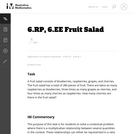Unrestricted Use
CC BY
Rating
0.0 stars

This is a task from the Illustrative Mathematics website that is one part of a complete illustration of the standard to which it is aligned. Each task has at least one solution and some commentary that addresses important asects of the task and its potential use. Here are the first few lines of the commentary for this task: A fruit salad consists of blueberries, raspberries, grapes, and cherries. The fruit salad has a total of 280 pieces of fruit. There are twice as many r...

Subject:
Mathematics
Material Type:
Activity/Lab
Provider:
Illustrative Mathematics
Provider Set:
Illustrative Mathematics
Author:
Illustrative Mathematics
05/15/2013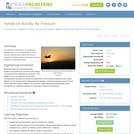Educational Use
Rating
0.0 stars

Air pressure is pushing on us all the time although we do not usually notice it. In this activity, students learn about the units of pressure and get a sense of just how much air pressure is pushing on them.

Subject:
Applied Science
Engineering
Physical Science
Physics
Material Type:
Activity/Lab
Provider:
TeachEngineering
Provider Set:
TeachEngineering
Author:
Alex Conner
Geoffrey Hill
Janet Yowell
Malinda Schaefer Zarske
Tom Rutkowski
10/14/2015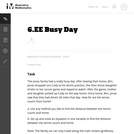Unrestricted Use
CC BY
Rating
0.0 stars

This task provides a good entry point for students into representing quantities in contexts with variables and expressions and building equations that reflect the relationships presented in the context.

Subject:
Mathematics
Material Type:
Activity/Lab
Provider:
Illustrative Mathematics
Provider Set:
Illustrative Mathematics
Author:
Illustrative Mathematics
08/15/2012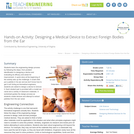Educational Use
Rating
0.0 stars

Students learn the engineering design process by following the steps, from problem identification to designing a device and evaluating its efficacy and areas for improvement. A quick story at the beginning of the activity sets up the challenge: A small child put a pebble in his ear and we don't know how to get it out! Acting as biomedical engineers, students are asked to design a device to remove it. Each student pair is provided with a model ear canal and a variety of classroom materials. A worksheet guides the design process as students create devices and attempt to extract pebbles from the ear canal.

Subject:
Applied Science
Engineering
Material Type:
Activity/Lab
Provider:
TeachEngineering
Provider Set:
TeachEngineering
Author:
Derek Harbin
Krista Warner
Leyf Starling
Shayn Peirce-Cottler
09/18/2014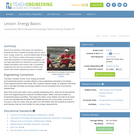Educational Use
Rating
0.0 stars

Demos and activities in this lesson are intended to illustrate the basic concepts of energy science -- work, force, energy, power etc. and the relationships among them. The "lecture" portion of the lesson includes many demonstrations to keep students engaged, yet has high expectations for the students to perform energy related calculations and convert units as required. A homework assignment and quiz are used to reinforce and assess these basic engineering science concepts.

Subject:
Applied Science
Engineering
Physical Science
Physics
Material Type:
Activity/Lab
Lesson Plan
Provider:
TeachEngineering
Provider Set:
TeachEngineering
Author:
Jan DeWaters
Susan Powers
09/18/2014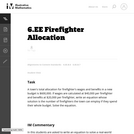Unrestricted Use
CC BY
Rating
0.0 stars

In this task students are asked to write an equation to solve a real-world problem. There are two natural approaches to this task. In the first approach, students have to notice that even though there is one variable, namely the number of firefighters, it is used in two different places. In the other approach, students can find the total cost per firefighter and then write the equation.

Subject:
Mathematics
Material Type:
Activity/Lab
Provider:
Illustrative Mathematics
Provider Set:
Illustrative Mathematics
Author:
Illustrative Mathematics
05/01/2012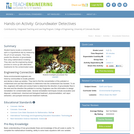Educational Use
Rating
0.0 stars

Student teams locate a contaminant spill in a hypothetical site by measuring the pH of soil samples. Then they predict the direction of groundwater flow using mathematical modeling. They also use the engineering design process to come up with alternative treatments for the contaminated water.

Subject:
Applied Science
Engineering
Environmental Science
Material Type:
Activity/Lab
Provider:
TeachEngineering
Provider Set:
TeachEngineering
Author:
Ben Heavner
Janet Yowell
Malinda Schaefer Zarske
Melissa Straten
10/14/2015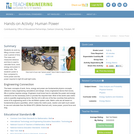Educational Use
Rating
0.0 stars

Students do work by lifting a known mass over a period of time. The mass and measured distance and time is used to calculate force, work, energy and power in metric units. The students' power is then compared to horse power and the power required to light 60-watt light bulbs.

Subject:
Applied Science
Engineering
Physical Science
Physics
Material Type:
Activity/Lab
Provider:
TeachEngineering
Provider Set:
TeachEngineering
Author:
Jan DeWaters
Susan Powers
09/18/2014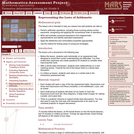Only Sharing Permitted
CC BY-NC-ND
Rating
0.0 stars

This lesson unit is intended to help you assess how well students are able to: Perform arithmetic operations, including those involving whole-number exponents, recognizing and applying the conventional order of operations; Write and evaluate numerical expressions from diagrammatic representations and be able to identify equivalent expressions; apply the distributive and commutative properties appropriately; and use the method for finding areas of compound rectangles.

Subject:
Geometry
Mathematics
Material Type:
Assessment
Lesson Plan
Provider:
Shell Center for Mathematical Education
Provider Set:
Mathematics Assessment Project (MAP)
04/26/2013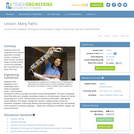Educational Use
Rating
0.0 stars

Students explore the composition and practical application of parallel circuitry, compared to series circuitry. Students design and build parallel circuits and investigate their characteristics, and apply Ohm's law.

Subject:
Applied Science
Career and Technical Education
Electronic Technology
Engineering
Material Type:
Activity/Lab
Lesson Plan
Provider:
TeachEngineering
Provider Set:
TeachEngineering
Author:
Daria Kotys-Schwartz
Denise Carlson
Janet Yowell
Malinda Schaefer Zarske
Sabre Duren
Xochitl Zamora Thompson
09/18/2014Conditional Remix & Share Permitted
CC BY-NC
Rating
0.0 stars
Rating
0.0 stars
Subject:
Mathematics
Material Type:
Full Course
Provider:
Pearson
10/06/2016Conditional Remix & Share Permitted
CC BY-NC
Rating
0.0 stars

Equations and Inequalities

Type of Unit: Concept

Prior Knowledge

Students should be able to:

Add, subtract, multiply, and divide with whole numbers, fractions, and decimals.
Use the symbols &lt;, &gt;, and =.
Evaluate expressions for specific values of their variables.
Identify when two expressions are equivalent.
Simplify expressions using the distributive property and by combining like terms.
Use ratio and rate reasoning to solve real-world problems.
Order rational numbers.
Represent rational numbers on a number line.

Lesson Flow

In the exploratory lesson, students use a balance scale to find a counterfeit coin that weighs less than the genuine coins. Then continuing with a balance scale, students write mathematical equations and inequalities, identify numbers that are, or are not, solutions to an equation or an inequality, and learn how to use the addition and multiplication properties of equality to solve equations. Students then learn how to use equations to solve word problems, including word problems that can be solved by writing a proportion. Finally, students connect inequalities and their graphs to real-world situations.

Subject:
Algebra
Mathematics
Material Type:
Unit of Study
Provider:
Pearson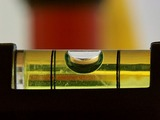Conditional Remix & Share Permitted
CC BY-NC
Rating
0.0 stars

Subject:
Algebra
Material Type:
Lesson Plan
09/21/2015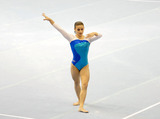Conditional Remix & Share Permitted
CC BY-NC
Rating
0.0 stars

Lesson OverviewStudents apply the multiplication property of equality to solve equations.Key ConceptsIn the previous lesson, students solved equations of the form x + p = q using the addition property of equality. In this lesson, they will solve equations of the form px = q using the multiplication property of equality. They will multiply or divide both sides of an equation by the same number to obtain an equivalent equation.Since multiplication by a is equivalent to division by 1a, students will see that they may also divide both sides of the equation by the same number to get an equivalent equation. Students will also apply this property to solving a particular kind of equation, a proportion.Goals and Learning ObjectivesUse the multiplication property of equality to keep an equation balanced.Use the multiplication property of equality to solve equations of the form px = q for cases in which p, q, and x are all non-negative rational numbers.Use the multiplication property of equality to solve proportions.

Subject:
Algebra
Material Type:
Lesson Plan
09/21/2015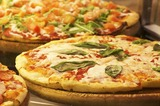Conditional Remix & Share Permitted
CC BY-NC
Rating
0.0 stars

Lesson OverviewStudents solve problems using equations of the form x + p = q and px = q, as well as problems involving proportions.Key ConceptsStudents will extend what they know about writing expressions to writing equations. An equation is a statement that two expressions are equivalent. Students will write two equivalent expressions that represent the same quantity. One expression will be numerical and the other expression will contain a variable.It is important that when students write the equation, they define the variable precisely. For example, n represents the number of minutes Aiko ran, or x represents the number of boxes on the shelf.Students will then solve the equations and thereby solve the problems.Students will solve proportion problems by solving equations. This makes sense because a proportion such as xa=bc is really just an equation of the form xp = q where p=1a and q=bc.Students will also compare their algebraic solutions to an arithmetic solution for the problem. They will see, for example, that a problem that might be solved arithmetically by subtracting 5 from 78 can also be solved algebraically by solving x + 5 = 78, where 5 is subtracted from both sides&mdash;a parallel solution to subtracting 5 from 78.Goals and Learning ObjectivesUse equations of the form x + p = q and xp = q to solve problems.Solve proportion problems using equations.ELL: ELLs may have difficulty verbalizing their reasoning, particularly because word problems are highly language dependent. Accommodate ELLs by providing extra time for them to process the information. Note that this problem is a good opportunity for ELLs to develop their literacy skills since it incorporates reading, writing, listening, and speaking skills. Encourage students to challenge each others&#39; ideas and justify their thinking using academic and specialized mathematical language.

Subject:
Algebra
Material Type:
Lesson Plan
09/21/2015Conditional Remix & Share Permitted
CC BY-NC
Rating
0.0 stars

Putting Math to Work

Type of Unit: Problem Solving

Prior Knowledge

Students should be able to:

Solve problems with rational numbers using all four operations.
Write ratios and rates.
Use a rate table to solve problems.
Write and solve proportions.
Use multiple representations (e.g., tables, graphs, and equations) to display data.
Identify the variables in a problem situation (i.e., dependent and independent variables).
Write formulas to show the relationship between two variables, and use these formulas to solve for a problem situation.
Draw and interpret graphs that show the relationship between two variables.
Describe graphs that show proportional relationships, and use these graphs to make predictions.
Interpret word problems, and organize information.
Graph in all quadrants of the coordinate plane.

Lesson Flow

As a class, students use problem-solving steps to work through a problem about lightning. In the next lesson, they use the same problem-solving steps to solve a similar problem about lightning. The lightning problems use both rational numbers and rates. Students then choose a topic for a math project. Next, they solve two problems about gummy bears using the problem-solving steps. They then have 3 days of Gallery problems to test their problem-solving skills solo or with a partner. Encourage students to work on at least one problem individually so they can better prepare for a testing situation. The unit ends with project presentations and a short unit test.

Subject:
Mathematics
Material Type:
Unit of Study
Provider:
PearsonConditional Remix & Share Permitted
CC BY-NC
Rating
0.0 stars

Students work in a whole-class setting, independently, and with partners to design and implement a problem-solving plan based on the mathematical concepts of rates and multiple representations (e.g., tables, equations, and graphs). They analyze a rule of thumb and use this relationship to calculate the distance in miles from a viewer&#39;s vantage point to lightning.Key ConceptsThroughout this unit, students are encouraged to apply the mathematical concepts they have learned over the course of this year to new settings. Help students develop and refine these problem-solving skills:Creating a problem-solving plan and implementing the plan systematicallyPersevering through challenging problems to find solutionsRecalling prior knowledge and applying that knowledge to new situationsMaking connections between previous learning and real-world problemsCommunicating their approaches with precision and articulating why their strategies and solutions are reasonableCreating efficacy and confidence in solving challenging problems in the real worldGoals and Learning ObjectivesCreate and implement a problem-solving plan.Organize and interpret data presented in a problem situation.Analyze the relationship between two variables.Create a rate table to organize data and make predictions.Apply the relationship between the variables to write a mathematical formula and use the formula to solve problems.Create a graph to display proportional relationships, and use this graph to make predictions.Articulate strategies, thought processes, and approaches to solving a problem, and defend why the solution is reasonable.

Subject:
Algebra
Material Type:
Lesson Plan
09/21/2015Conditional Remix & Share Permitted
CC BY-NC
Rating
0.0 stars

During this two-day lesson, students work with a partner to create and implement a problem-solving plan based on the mathematical concepts of rates, ratios, and proportionality. Students analyze the relationship between different-sized gummy bears to solve problems involving size and price.Key ConceptsThroughout this unit, students are encouraged to apply the mathematical concepts they have learned over the course of this year to new settings. Help students develop and refine these problem-solving skills:Creating a problem solving plan and implementing their plan systematicallyPersevering through challenging problems to find solutionsRecalling prior knowledge and applying that knowledge to new situationsMaking connections between previous learning and real-world problemsCommunicating their approaches with precision and articulating why their strategies and solutions are reasonableCreating efficacy and confidence in solving challenging problems in a real worldGoals and Learning ObjectivesCreate and implement a problem-solving plan.Organize and interpret data presented in a problem situation.Analyze the relationship between two variables.Use ratios.Write and solve proportions.Create rate tables to organize data and make predictions.Use multiple representations&mdash;including tables, graphs, and equations&mdash;to organize and communicate data.Articulate strategies, thought processes, and approaches to solving a problem, and defend why the solution is reasonable.

Subject:
Ratios and Proportions
Material Type:
Lesson Plan